Anúncio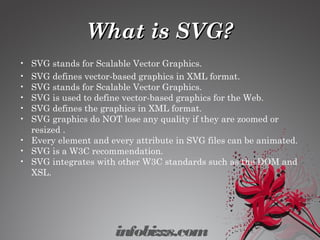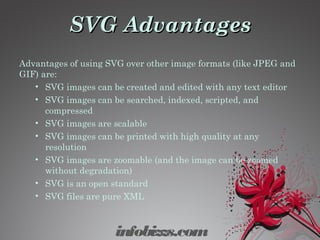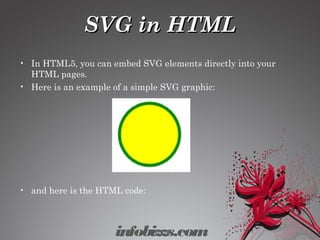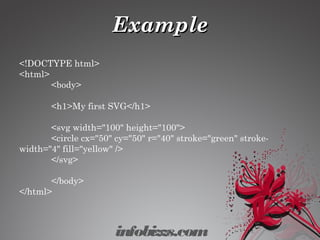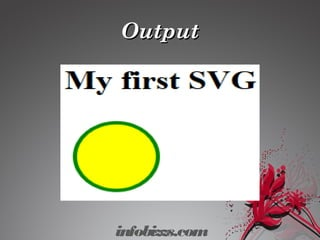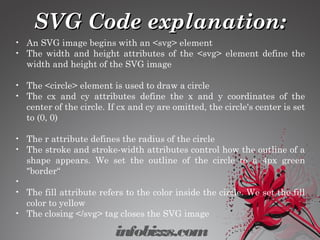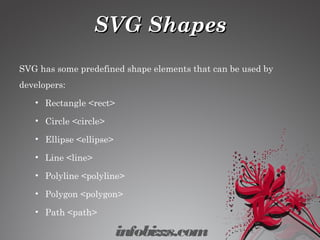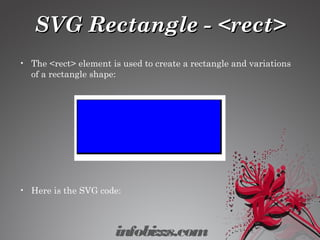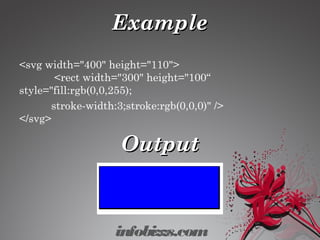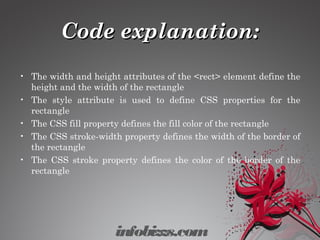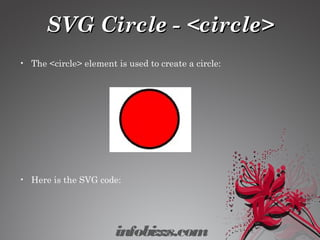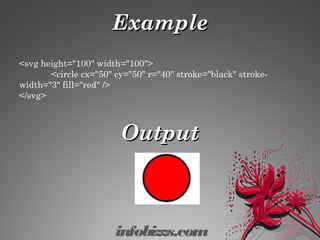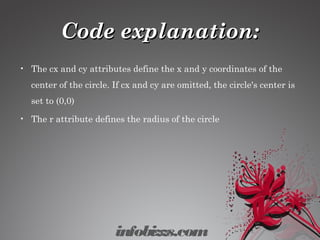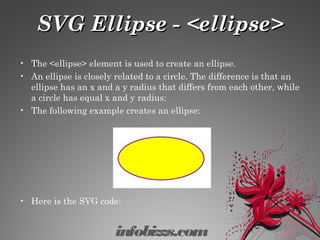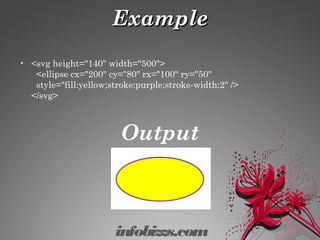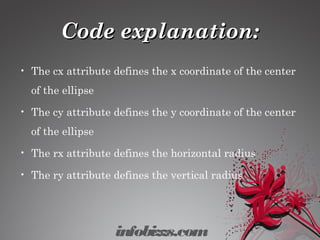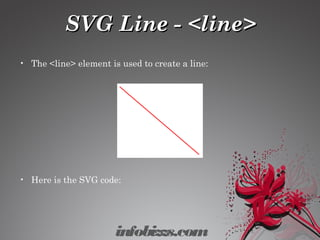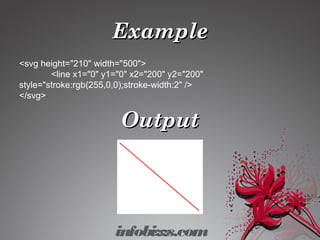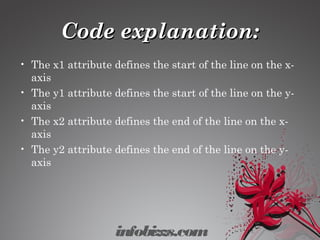1 de 20
Anúncio

### Svg

1. SVG(ScalableSVG(Scalable Vector Graphics)Vector Graphics) infobizzs.com
2. What is SVG?What is SVG? • SVG stands for Scalable Vector Graphics. • SVG defines vector-based graphics in XML format. • SVG stands for Scalable Vector Graphics. • SVG is used to define vector-based graphics for the Web. • SVG defines the graphics in XML format. • SVG graphics do NOT lose any quality if they are zoomed or resized . • Every element and every attribute in SVG files can be animated. • SVG is a W3C recommendation. • SVG integrates with other W3C standards such as the DOM and XSL. infobizzs.com
3. SVG AdvantagesSVG Advantages Advantages of using SVG over other image formats (like JPEG and GIF) are: • SVG images can be created and edited with any text editor • SVG images can be searched, indexed, scripted, and compressed • SVG images are scalable • SVG images can be printed with high quality at any resolution • SVG images are zoomable (and the image can be zoomed without degradation) • SVG is an open standard • SVG files are pure XML infobizzs.com
4. SVG in HTMLSVG in HTML • In HTML5, you can embed SVG elements directly into your HTML pages. • Here is an example of a simple SVG graphic: • and here is the HTML code: infobizzs.com
5. ExampleExample <!DOCTYPE html> <html> <body> <h1>My first SVG</h1> <svg width="100" height="100">   <circle cx="50" cy="50" r="40" stroke="green" stroke- width="4" fill="yellow" /> </svg> </body> </html> infobizzs.com
6. OutputOutput infobizzs.com
7. SVG Code explanation:SVG Code explanation: infobizzs.com • An SVG image begins with an <svg> element • The width and height attributes of the <svg> element define the width and height of the SVG image • The <circle> element is used to draw a circle • The cx and cy attributes define the x and y coordinates of the center of the circle. If cx and cy are omitted, the circle's center is set to (0, 0) • The r attribute defines the radius of the circle • The stroke and stroke-width attributes control how the outline of a shape appears. We set the outline of the circle to a 4px green "border“ • • The fill attribute refers to the color inside the circle. We set the fill color to yellow • The closing </svg> tag closes the SVG image
8. SVG ShapesSVG Shapes SVG has some predefined shape elements that can be used by developers: • Rectangle <rect> • Circle <circle> • Ellipse <ellipse> • Line <line> • Polyline <polyline> • Polygon <polygon> • Path <path> infobizzs.com
9. SVG Rectangle - <rect>SVG Rectangle - <rect> • The <rect> element is used to create a rectangle and variations of a rectangle shape: • Here is the SVG code: infobizzs.com
10. ExampleExample <svg width="400" height="110">   <rect width="300" height="100“ style="fill:rgb(0,0,255); stroke-width:3;stroke:rgb(0,0,0)" /> </svg> OutputOutput infobizzs.com
11. Code explanation:Code explanation: • The width and height attributes of the <rect> element define the height and the width of the rectangle • The style attribute is used to define CSS properties for the rectangle • The CSS fill property defines the fill color of the rectangle • The CSS stroke-width property defines the width of the border of the rectangle • The CSS stroke property defines the color of the border of the rectangle infobizzs.com
12. SVG Circle - <circle>SVG Circle - <circle> • The <circle> element is used to create a circle: • Here is the SVG code: infobizzs.com
13. ExampleExample <svg height="100" width="100">   <circle cx="50" cy="50" r="40" stroke="black" stroke- width="3" fill="red" /> </svg> OutputOutput infobizzs.com
14. Code explanation:Code explanation: • The cx and cy attributes define the x and y coordinates of the center of the circle. If cx and cy are omitted, the circle's center is set to (0,0) • The r attribute defines the radius of the circle infobizzs.com
15. SVG Ellipse - <ellipse>SVG Ellipse - <ellipse> • The <ellipse> element is used to create an ellipse. • An ellipse is closely related to a circle. The difference is that an ellipse has an x and a y radius that differs from each other, while a circle has equal x and y radius: • The following example creates an ellipse: • Here is the SVG code: infobizzs.com
16. ExampleExample • <svg height="140" width="500">   <ellipse cx="200" cy="80" rx="100" ry="50"   style="fill:yellow;stroke:purple;stroke-width:2" /> </svg> Output infobizzs.com
17. Code explanation:Code explanation: • The cx attribute defines the x coordinate of the center of the ellipse • The cy attribute defines the y coordinate of the center of the ellipse • The rx attribute defines the horizontal radius • The ry attribute defines the vertical radius infobizzs.com
18. SVG Line - <line>SVG Line - <line> • The <line> element is used to create a line: • Here is the SVG code: infobizzs.com
19. ExampleExample <svg height="210" width="500"> <line x1="0" y1="0" x2="200" y2="200" style="stroke:rgb(255,0,0);stroke-width:2" /> </svg> OutputOutput infobizzs.com
20. Code explanation:Code explanation: • The x1 attribute defines the start of the line on the x- axis • The y1 attribute defines the start of the line on the y- axis • The x2 attribute defines the end of the line on the x- axis • The y2 attribute defines the end of the line on the y- axis infobizzs.com
Anúncio